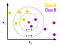# Chapter 4: K Nearest Neighbors Classifier

he fourth and last basic classifier in supervised learning! K nearest Neighbors. In this post, we will discuss about working of K Nearest Neighbors Classifier, the three different underlying algorithms for choosing a neighbor and a part of code snippet for python’s sklearn library. If you haven’t read the previous posts on:

1. Naive Bayes
2. SVM (Support Vector)
3. Decision Trees

I would suggest you to go through it prior to this one. (Although this is independent from those three)

# In Short,

An object is classified by a majority vote of its neighbors, with the object being assigned to the class most common among its k nearest neighbors (k is a positive integer, typically small). If k = 1, then the object is simply assigned to the class of that single nearest neighbor.class of star would be B if k =3 and A if k = 6

# How do we choose neighbors?

## Brute Force

Lets consider for simple case with two dimension plot. If we look mathematically, the simple intuition is to calculate the euclidean distance from point of interest ( of whose class we need to determine) to all the points in training set. Then we take class with majority points. This is called brute force method.

For N samples in D dimensions the running time complexity turns out to be O[DN²]. If we have small number of dimensions and training set, this would run in reasonable time. But as the training set size increases, the running time grows quickly.

Brute force performs worst when there are large dimensions and large training set.

## K-D Tree

To improve the running time, alternate approaches were invented on the line of building a growing tree from point of interest. These methods attempt to reduce the required number of distance calculations by efficiently encoding aggregate distance information for the sample.

The basic idea is that if point A is very distant from point B, and point B is very close to point C, then we know that points A and C are very distant, without having to explicitly calculate their distance. (This may not be correct all the time.)

In this way, the computational cost of a nearest neighbors search can be reduced to O[D N *log(N)] or better. This is a significant improvement over brute-force for large N.

K-D tree performs well enough when D <20. With larger D it again takes longer time. This is known as “curse of dimensionality”.

## Curse of Dimensionality explained to Kid [Source : StackOverflow]

Probably the kid will like to eat cookies, so let us assume that you have a whole truck with cookies having a different color, a different shape, a different taste, a different price …

If the kid has to choose but only take into account one characteristic e.g. the taste, then it has four possibilities: sweet, salt, sour, bitter, so the kid only has to try four cookies to find what (s)he likes most.

If the kid likes combinations of taste and colour, and there are 4 (I am rather optimistic here :-) ) different colours, then he already has to choose among 4x4 different types;

If he wants, in addition, to take into account the shape of the cookies and there are 5 different shapes then he will have to try 4x4x5=80 cookies

We could go on, but after eating all these cookies he might already have belly-ache … before he can make his best choice :-) Apart from the belly-ache, it can get really difficult to remember the differences in the taste of each cookie.

As you can see complexity increases when dimensions/features increases.

## Ball Tree

Ball tree assumes the data in multidimensional dimensional space and creates the nested hyper spheres. Query Time complexity is O[Dlog(N)].

# Which one to choose when?

The selection of the relevant algorithm for problem at hand depends on the number of dimensions and the size of training set.

1. For small sample size and small dimensions, brute force performs well.
2. Sparsity of data : If data is sparse with small dimensions (< 20) KD tree will perform better than Ball Tree algorithm.
3. Value of K (neighbors) : As the K increases, query time of both KD tree and Ball tree increases.

# Sklearn K nearest and parameters

Sklearn in python provides implementation for K Nearest Neighbors Classifier. Below is sample code snippet to use in python:

`from sklearn.neighbors import KNeighborsClassifierneigh = KNeighborsClassifier(n_neighbors=3)neigh.fit(features_matrix, labels)predicted_values = neigh.predict(test_matrix)`

## Some important Parameters

n_neighbors : Number of neighbors to consider. Default is 5.

algorithm: By default it is set to auto. Algorithm can be {‘auto’, ‘ball_tree’, ‘kd_tree’, ‘brute’}.

Currently, algorithm = ‘auto’ selects ‘kd_tree’ if k < N/2 and the ‘effective_metric_’ is in the ‘VALID_METRICS’ list of ‘kd_tree’. It selects ‘ball_tree’ if k < N/2 and the ‘effective_metric_’ is not in the ‘VALID_METRICS’ list of ‘kd_tree’. It selects ‘brute’ if k >= N/2. This choice is based on the assumption that the number of query points is at least the same order as the number of training points, and that leaf_size is close to its default value of 30. [ reference : Sklearn Documentation].

You can explore all the parameters here.

# Final Thoughts

Except brute force, K Nearest Neighbors implements tree like data structure to determine the distances from point of interest to points in training set. The selection of best algorithm depends on sparsity of data, number of neighbors requested and the dimension/number of features.

I hope that this section was helpful in understanding the working behind K Nearest Neighbor classifier. Comment down your thoughts, feedback or suggestions if any below. If you liked this post, share it with your friends, subscribe to Machine Learning 101 click the heart(❤) icon. Follow me on Facebook, twitter, LinkedIn. Peace!

Written by